# High School Chemistry : Balancing Oxidation-Reduction Reactions

## Example Questions

### Example Question #1 : Balancing Oxidation Reduction Reactions

What is the coefficient on sulfur dioxide if the following redox reaction is balanced in an acidic solution?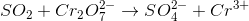Possible Answers:Correct answer:Explanation:

Balancing redox reactions involves the following steps:

1. Divide the reaction into oxidation and reduction half reactions and balance all atoms that are not oxygen and hydrogen: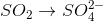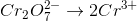2. Balance the oxygens by adding water molecules on the opposite side of the reactions: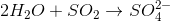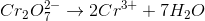3. Balance the hydrogens by adding protons to the opposite side of the equation: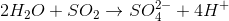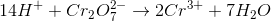4. Add electrons in order to equal the charges on both sides of the equation: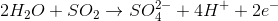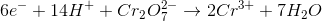5. In order to make the electron exchange equal in each half step, we must multiply the top half reaction by 3: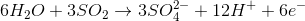6. Add up the reactants and products while cancelling out substances on opposite sides of the reactions. For example: we will cancel the 6 water molecules as reactants and be left with only one water molecule as a product. In addition, only 2 protons will be left on the reactant's side after canceling the 12 from the product's side.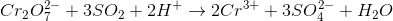In the balanced redox reaction, the coefficient on sulfur dioxide is 3.

### Example Question #2 : Balancing Oxidation Reduction Reactions

For the following unbalanced redox reaction, how many electrons are transferred and which chemical species is being oxidized?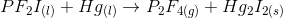Possible Answers:

One electron is transferred; Hg is oxidized

Two electrons are transferred; Hg is oxidized

One electron is transferred; P is oxidized

Two electrons are transferred; P is oxidized

Correct answer:

Two electrons are transferred; Hg is oxidized

Explanation:

To begin, we will need to separate the given reaction into the two half-reactions by identifying changes in oxidation number. In this case, mercury (Hg) and phosphorus (P) show a change in oxidation number. Mercury begins with an oxidation number of zero, and ends with an oxidation number of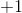. Phosphorus begins with an oxidation number of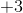and ends with an oxidation number of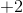. Note that the oxidation numbers for fluorine and iodine reamain constant atfor each.

Now we can begin to look at the half-reactions.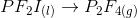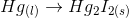Balance the atoms.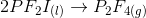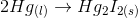Now balance the electrons. We know that each mercury atom loses one electron and each fluorine atom gains one electron.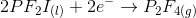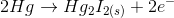We can see that two electrons are tranferred. To identify the element being oxidized, we must find the element that is losing electrons. In this case, mercury is being oxidized.

### Example Question #2 : Balancing Oxidation Reduction Reactions

Oxidation is the __________ of electrons, reduction is the __________ of electrons.

Possible Answers:

gain . . . loss

loss . . . gain

None of these; it depends on the reaction.

loss . . . loss

gain . . . gain

Correct answer:

loss . . . gain

Explanation:

An oxidation-reduction (redox) reaction is a reaction where electrons are transferred between two substances. When an atom is oxidized, it is called the reducing agent, and it loses a number of electrons. Similarly, a reduced atom is called the oxidizing agent, and gains the same number of electrons. A popular mnemonic to help remember is OIL RIG, or Oxidation is Loss of electrons, Reduction is Gain of electrons.

### All High School Chemistry Resources